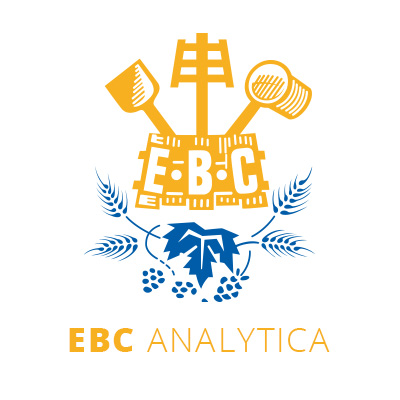Analytica EBC | Statistics | 14.2 - Collaborative Trial to Determine the Precision of a Measurement Method
CONTACT |  info@brewup.eu

# ANALYTICA EBC### 14.2 - Collaborative Trial to Determine the Precision of a Measurement Method

• 24/10/2018
• 2095
• |
• Statistics
• |
• 2002

Providing an aid to the correct understanding of the results of precision experiments and of the terminology employed. Descriptors: the true value, the laboratory component of bias under repeatability conditions, the random error occurring in every measurement under repeatability conditions, the estimate of the variance of B (between laboratory variance), the estimate of the repeatability variance (vatiance of e), the estimate of the reproducibility variance, the first test result of laboratory i at level j, the second test result of laboratory i at level j, the general mean for level j, The reproducibility variance for the j level, The repeatability limit at the 95 % probability level, The reproducibility limit at the 95 % probability level, the number of laboratories reporting at least one test result for level j, the intracell standard deviation calculated with the formula given in 7.1.4, the mean of all observations, the standard deviation of the observations.

It is necessary to use EBC Method 14.1

### Related documents24/10/2018
Statistics